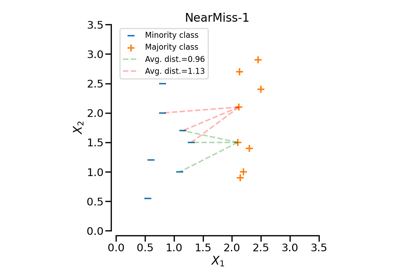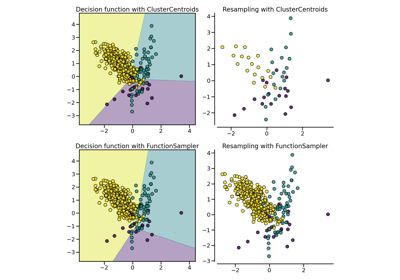# NearMiss#

class imblearn.under_sampling.NearMiss(*, sampling_strategy='auto', version=1, n_neighbors=3, n_neighbors_ver3=3, n_jobs=None)[source]#

Class to perform under-sampling based on NearMiss methods.

Read more in the User Guide.

Parameters
sampling_strategyfloat, str, dict, callable, default=’auto’

Sampling information to sample the data set.

• When float, it corresponds to the desired ratio of the number of samples in the minority class over the number of samples in the majority class after resampling. Therefore, the ratio is expressed as $$\alpha_{us} = N_{m} / N_{rM}$$ where $$N_{m}$$ is the number of samples in the minority class and $$N_{rM}$$ is the number of samples in the majority class after resampling.

Warning

float is only available for binary classification. An error is raised for multi-class classification.

• When str, specify the class targeted by the resampling. The number of samples in the different classes will be equalized. Possible choices are:

'majority': resample only the majority class;

'not minority': resample all classes but the minority class;

'not majority': resample all classes but the majority class;

'all': resample all classes;

'auto': equivalent to 'not minority'.

• When dict, the keys correspond to the targeted classes. The values correspond to the desired number of samples for each targeted class.

• When callable, function taking y and returns a dict. The keys correspond to the targeted classes. The values correspond to the desired number of samples for each class.

versionint, default=1

Version of the NearMiss to use. Possible values are 1, 2 or 3.

n_neighborsint or estimator object, default=3

If int, size of the neighbourhood to consider to compute the average distance to the minority point samples. If object, an estimator that inherits from KNeighborsMixin that will be used to find the k_neighbors. By default, it will be a 3-NN.

n_neighbors_ver3int or estimator object, default=3

If int, NearMiss-3 algorithm start by a phase of re-sampling. This parameter correspond to the number of neighbours selected create the subset in which the selection will be performed. If object, an estimator that inherits from KNeighborsMixin that will be used to find the k_neighbors. By default, it will be a 3-NN.

n_jobsint, default=None

Number of CPU cores used during the cross-validation loop. None means 1 unless in a joblib.parallel_backend context. -1 means using all processors. See Glossary for more details.

Attributes
sampling_strategy_dict

Dictionary containing the information to sample the dataset. The keys corresponds to the class labels from which to sample and the values are the number of samples to sample.

nn_estimator object

Validated K-nearest Neighbours object created from n_neighbors parameter.

sample_indices_ndarray of shape (n_new_samples,)

Indices of the samples selected.

New in version 0.4.

n_features_in_int

Number of features in the input dataset.

New in version 0.9.

feature_names_in_ndarray of shape (n_features_in_,)

Names of features seen during fit. Defined only when X has feature names that are all strings.

New in version 0.10.

RandomUnderSampler

Random undersample the dataset.

InstanceHardnessThreshold

Use of classifier to undersample a dataset.

Notes

The methods are based on .

Supports multi-class resampling.

References

1

I. Mani, I. Zhang. “kNN approach to unbalanced data distributions: a case study involving information extraction,” In Proceedings of workshop on learning from imbalanced datasets, 2003.

Examples

>>> from collections import Counter
>>> from sklearn.datasets import make_classification
>>> from imblearn.under_sampling import NearMiss
>>> X, y = make_classification(n_classes=2, class_sep=2,
... weights=[0.1, 0.9], n_informative=3, n_redundant=1, flip_y=0,
... n_features=20, n_clusters_per_class=1, n_samples=1000, random_state=10)
>>> print('Original dataset shape %s' % Counter(y))
Original dataset shape Counter({1: 900, 0: 100})
>>> nm = NearMiss()
>>> X_res, y_res = nm.fit_resample(X, y)
>>> print('Resampled dataset shape %s' % Counter(y_res))
Resampled dataset shape Counter({0: 100, 1: 100})


Methods

 fit(X, y) Check inputs and statistics of the sampler. fit_resample(X, y) Resample the dataset. get_feature_names_out([input_features]) Get output feature names for transformation. get_params([deep]) Get parameters for this estimator. set_params(**params) Set the parameters of this estimator.
fit(X, y)[source]#

Check inputs and statistics of the sampler.

You should use fit_resample in all cases.

Parameters
X{array-like, dataframe, sparse matrix} of shape (n_samples, n_features)

Data array.

yarray-like of shape (n_samples,)

Target array.

Returns
selfobject

Return the instance itself.

fit_resample(X, y)[source]#

Resample the dataset.

Parameters
X{array-like, dataframe, sparse matrix} of shape (n_samples, n_features)

Matrix containing the data which have to be sampled.

yarray-like of shape (n_samples,)

Corresponding label for each sample in X.

Returns
X_resampled{array-like, dataframe, sparse matrix} of shape (n_samples_new, n_features)

The array containing the resampled data.

y_resampledarray-like of shape (n_samples_new,)

The corresponding label of X_resampled.

get_feature_names_out(input_features=None)[source]#

Get output feature names for transformation.

Parameters
input_featuresarray-like of str or None, default=None

Input features.

• If input_features is None, then feature_names_in_ is used as feature names in. If feature_names_in_ is not defined, then the following input feature names are generated: ["x0", "x1", ..., "x(n_features_in_ - 1)"].

• If input_features is an array-like, then input_features must match feature_names_in_ if feature_names_in_ is defined.

Returns
feature_names_outndarray of str objects

Same as input features.

get_params(deep=True)[source]#

Get parameters for this estimator.

Parameters
deepbool, default=True

If True, will return the parameters for this estimator and contained subobjects that are estimators.

Returns
paramsdict

Parameter names mapped to their values.

set_params(**params)[source]#

Set the parameters of this estimator.

The method works on simple estimators as well as on nested objects (such as Pipeline). The latter have parameters of the form <component>__<parameter> so that it’s possible to update each component of a nested object.

Parameters
**paramsdict

Estimator parameters.

Returns
selfestimator instance

Estimator instance.

## Examples using imblearn.under_sampling.NearMiss#Multiclass classification with under-sampling

Multiclass classification with under-samplingSample selection in NearMiss

Sample selection in NearMissCompare under-sampling samplers

Compare under-sampling samplers